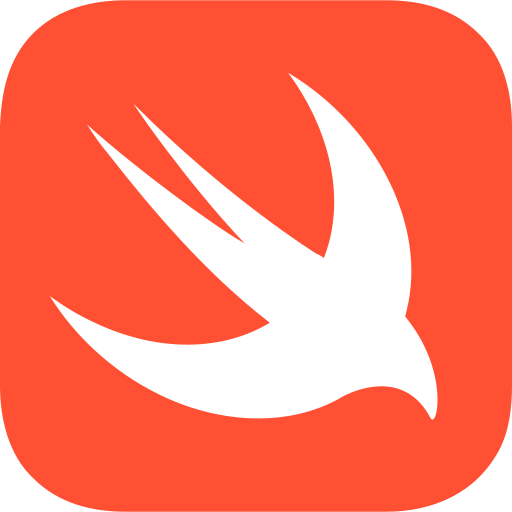# AI coding assistant for busy programmers

## How to check if the given number is a prime number in Swift## 🤖 Code Explanation

isPrime is a function that takes an integer as input and returns a boolean value. The function checks if the number is a prime number or not. If the number is less than or equal to 1, it returns false. If the number is less than or equal to 3, it returns true. Otherwise, the function checks if the number is divisible by any number between 2 and the square root of the number. If the number is not divisible by any number between 2 and the square root of the number, it returns true. Otherwise, it returns false.